# High School Chemistry : Balancing Reactions

## Example Questions

1 3 Next →

### Example Question #12 : Balancing Chemical Equations

Which of the following sets of coefficients correctly balance the following chemical reaction:

__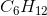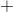__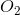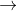__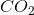__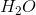?

Possible Answers:, and, and, and, and, andCorrect answer:, andExplanation:

One molecule of, balances with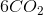molecules and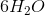molecules. On the right side of the equation, there are a total of 18 oxygen atoms, which equates to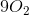molecules on the left side of the equation.

### Example Question #12 : Balancing Chemical Equations

What are the coefficients for each species when the chemical reaction shown is balanced?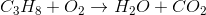Possible Answers:

1,3,2,6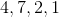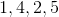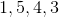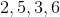Correct answer:Explanation:

As when balancing the vast majority of chemical reaction, leave oxygen as the last element to balance. Start by balancing the hydrogen atoms - this requires a 4 in front of water on the products side. Next, balance the three carbon atoms on the reactant side by adding a coefficient of 3 to the carbon dioxide in the products. Finally balance the oxygen atoms by placing a 5 in front of the oxygen gas on the reactant side. Double check to make sure there is a 1:1 ratio of each element and that all coefficients are whole numbers. Thus the balanced chemical reaction is: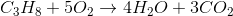### Example Question #14 : Balancing Chemical Equations

When the following equation is balanced, what is the coefficient in front of aluminum?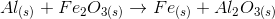Possible Answers:Correct answer:Explanation:Balance aluminum by adding a coefficient of 2 to the left side: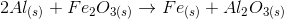Balance iron by adding a coefficient of 2 to the right side: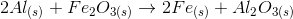The equation is balanced.

### Example Question #21 : Balancing Chemical Equations

Balance the following equation: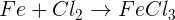Possible Answers: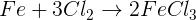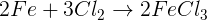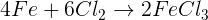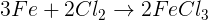Correct answer:Explanation:

When balancing a chemical equation, it means that we are adjusting the coefficients in front of every compound so that the number of atoms for every element will match how many will be on the other side of the arrow.Initially, we must take "inventory" of how many atoms of each element we have on both sides of the arrow.

On the left side:

1 iron, 2 chlorine

On the right side:

1 iron, 3 chlorine

While the iron atoms are balanced, the chlorine atoms are not. Now we must think, what is the least common multiple of 2 and 3? We can quickly realize it is 6. So we must add a coefficient in front of every term that includes a chlorine so we may make it 6 chlorine atoms on both sides of the arrow. (The coefficient will be multiplied by the subscript attached to the chlorine atom.)While the chlorine atoms are balanced now, we have lost the balance for the iron atoms. This can be quickly resolved. On the left, we still only have one iron atom. On the right, it has changed to 2 iron atoms. This means that in order to balance the number of iron atoms, we just need to add a 2 coefficient in front of the iron on the left side of the arrow.Now let's do one last tally to make sure we've balanced everything.

On the left:

2 iron, 6 chlorine

On the right:

2 iron, 6 chlorine

The equation has been balanced.

### Example Question #21 : Balancing Reactions

Balance the following equation: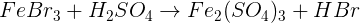Possible Answers: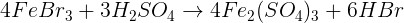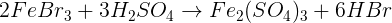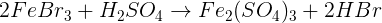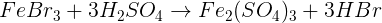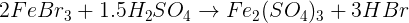Correct answer:Explanation:

When balancing a chemical equation, it means that we are adjusting the coefficients in front of every compound so that the number of atoms for every element will match how many will be on the other side of the arrow.Initially, we must take "inventory" of how many atoms of each element we have on both sides of the arrow.

On the left side:

1 iron, 3 bromine, 2 hydrogen, 1 sulfur, 4 O

On the right side:

2 iron, 1 bromine, 1 hydrogen, 3 sulfur, 12 O

Since there are quite a few components in this example, all of which need balancing, we just need to choose one element to start with and continue from there. Let's look at iron. Since there is only one iron atom on the left side, and 2 on the right side, let's think about what the lowest common multiple between 1 and 2 is - we can quickly decide that it is 2. This means we may add a 2 coefficient in front of the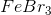on the left side of the arrow. (The coefficient is multiplied by the subscript of the atom - in this case, 2 multiplies by 1.)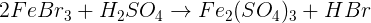Adding the 2 coefficient in front ofchanges how many bromine atoms we have on the left side to 6 atoms from the original 3. Comparing to the right side, which has 1 bromine atom, we can realize the lowest common multiple 6 and 1 share is 6. This means we must place a 6 coefficient in front of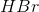on the right side of the arrow.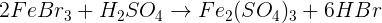By placing that 6, we change the number of hydrogen atoms on the right side to 6 from 1. Comparing that to number of hydrogen atoms on the left side of the arrow (2), we can determine that the lowest common multiple between 6 and 2 is 6. This means that we must place a 3 coefficient in front of the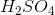on the left hand side.By placing that last coefficient, this affected the number of sulfur and oxygen atoms on the left side - we now have 3 sulfur atoms and 12 oxygen atoms on the left side. Comparing this to the right side, where we also have 3 sulfur atoms and 12 oxygen atoms. So this last step has balanced the rest of our atoms!

Now let's do one last tally to make sure we've balanced everything.

On the left:

2 iron, 6 bromine, 6 hydrogen, 3 sulfur and 12 oxygen

On the right:

2 iron, 6 bromine, 6 hydrogen, 3 sulfur and 12 oxygen

The equation has been balanced.

### Example Question #21 : Balancing Chemical Equations

Balance the following equation: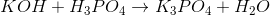Possible Answers: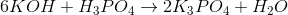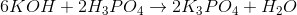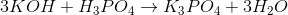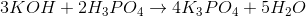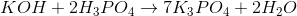Correct answer:Explanation:

When balancing a chemical equation, it means that we are adjusting the coefficients in front of every compound so that the number of atoms for every element will match how many will be on the other side of the arrow.Initially, we must take "inventory" of how many atoms of each element we have on both sides of the arrow.

On the left side:

1 potassium, 5 oxygen, 4 hydrogen, 1 P

On the right side:

3 potassium, 5 oxygen, 2 hydrogen, 1 P

Since there are quite a few components in this example, all of which need balancing, we just need to choose one element to start with and continue from there. Let's look at potassium. Since there is only one potassium atom on the left side, and 3 on the right side, let's think about what the lowest common multiple between 1 and 3 is - we can quickly decide that it is 3. This means we may place a 3 coefficient in front of the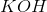on the left side of the arrow. (The coefficient is multiplied by the subscript of the atom - in this case, 3 multiplies by 1.)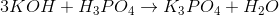Adding the 3 coefficient in front ofchanges how many oxygen and hydrogen atoms we have on the left side to 7 oxygen atoms and  6 hydrogen atoms. Comparing to the right side, which has 5 oxygen atoms and 2 hydrogen atoms. Since balancing the oxygen atoms on both sides is slightly more complicated because oxygen appears in two compounds, we will not manipulate their numbers but let their numbers become balanced through balancing the atoms around them. So let's turn our attention to the hydrogen atoms. If we have 6 hydrogen atoms on the left side, and 2 hydrogen atoms on the right (in one compound), we can come to terms with 6 being the lowest common multiple for 6 and 2. This means we need to place a 3 coefficient in front ofon the right side.This brings our total of oxygen atoms on the right side to 7 as well. So we have indirectly balanced the oxygen atoms. Since the only element left to balance is phosphorus, we realize that phosphorus on both sides is already balanced. We have balanced our equation.

Now let's do one last tally to make sure we've balanced everything.

On the left:

3 potassium, 7 oxygen, 6 hydrogen, 1 phosphorus

On the right:

3 potassium, 7 oxygen, 6 hydrogen, 1 phosphorus

The equation has been balanced.

1 3 Next →

### All High School Chemistry Resources